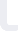Matlab: intermediate course for engineers and STEM-students Udemy

# Matlab: intermediate course for engineers and STEM-students هذه الدورة تدرس باللغة الإنجليزية

## Udemy

السعر: 95 USD

### تفاصيل الدورة

This is an intermediate Matlab course for engineering and STEM-students and professionals in these areas. The course is focussing on two main topics: usage of symbolic variables and expressions and solving different kinds of equations in Matlab. Differential equations play a major role in engineering and natural sciences and this course is going to provide you the knowledge how Matlab can help you soling such equations (or systems) straigth-forward and numerically in Matlab.

تحديث بتاريخ 22 March, 2018
دورات يمكنك الالتحاق بها على الفور... خذ دورة عبر الإنترنت على MATLAB ابتداءً من الآن.
See all courses